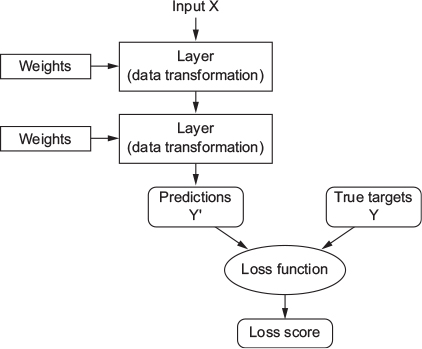## 15.8 Understanding how DL works (2)

• Loss function (also called objective function)
• To control output of neural network, you need to be able to measure how far this output is from what you expected
• Loss function takes predictions of the network and the true target (what you wanted the network to output) and computes a distance score, capturing how well the network has done on this specific example (see Figure 15.5).Figure 15.5: A loss function measures the quality of the network’s output (Chollet & Allaire, 2018, Fig. 1.8)